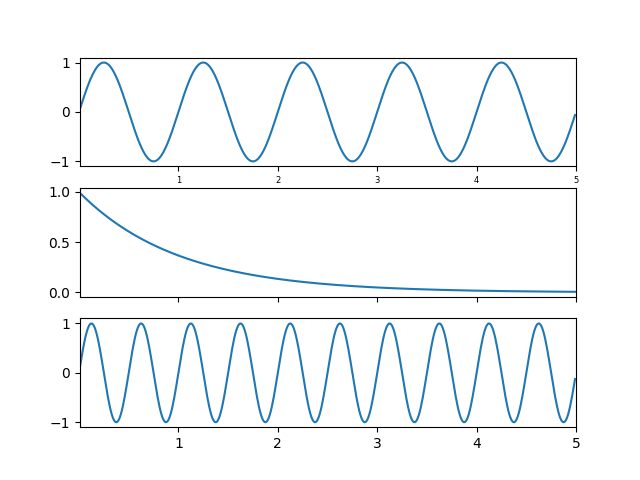# Shared Axis Demo¶

You can share the x or y axis limits for one axis with another by passing an axes instance as a sharex or sharey kwarg.

Changing the axis limits on one axes will be reflected automatically in the other, and vice-versa, so when you navigate with the toolbar the axes will follow each other on their shared axes. Ditto for changes in the axis scaling (e.g., log vs linear). However, it is possible to have differences in tick labeling, e.g., you can selectively turn off the tick labels on one axes.

The example below shows how to customize the tick labels on the various axes. Shared axes share the tick locator, tick formatter, view limits, and transformation (e.g., log, linear). But the ticklabels themselves do not share properties. This is a feature and not a bug, because you may want to make the tick labels smaller on the upper axes, e.g., in the example below.

If you want to turn off the ticklabels for a given axes (e.g., on subplot(211) or subplot(212), you cannot do the standard trick:

```setp(ax2, xticklabels=[])
```

because this changes the tick Formatter, which is shared among all axes. But you can alter the visibility of the labels, which is a property:

```setp(ax2.get_xticklabels(), visible=False)
``````import matplotlib.pyplot as plt
import numpy as np

t = np.arange(0.01, 5.0, 0.01)
s1 = np.sin(2 * np.pi * t)
s2 = np.exp(-t)
s3 = np.sin(4 * np.pi * t)

ax1 = plt.subplot(311)
plt.plot(t, s1)
plt.setp(ax1.get_xticklabels(), fontsize=6)

# share x only
ax2 = plt.subplot(312, sharex=ax1)
plt.plot(t, s2)
# make these tick labels invisible
plt.setp(ax2.get_xticklabels(), visible=False)

# share x and y
ax3 = plt.subplot(313, sharex=ax1, sharey=ax1)
plt.plot(t, s3)
plt.xlim(0.01, 5.0)
plt.show()
```

Keywords: matplotlib code example, codex, python plot, pyplot Gallery generated by Sphinx-Gallery